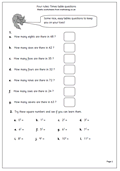# Times tables and square numbersRather a mixed bag of questions here. The first half asks questions derived from the times tables, such as ‘How many fours in 32?’ This is an important question to ask before moving on to the long method of division.

The second set of questions looks at square numbers, which, of course, give a diagonal pattern on a tables square. Children need to be familiar with the square sign to tackle this. It is extremely useful to know, off by heart, all the square numbers, up to at least 10 x 10, as it will be a great help later in High School.

This page can be found in our Four Rules section, under Multiplication (mental methods).

Times table questions (pg 1)